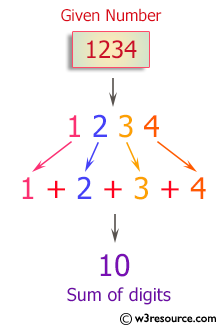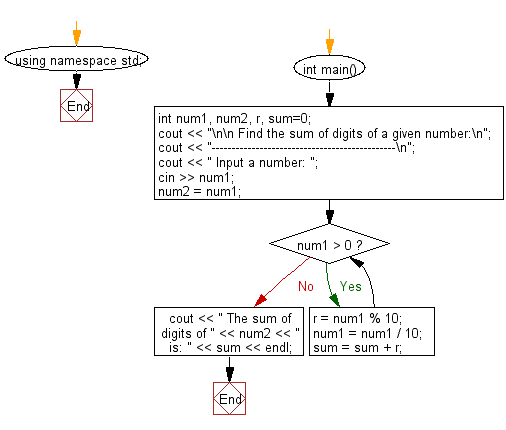﻿ C++ Exercises: Find the sum of digits of a given number - w3resource

# C++ Exercises: Find the sum of digits of a given number

## C++ For Loop: Exercise-10 with Solution

Write a program in C++ to find the sum of the digits of a given number.

Pictorial Presentation:Sample Solution :-

C++ Code :

``````#include <iostream>
using namespace std;
int main()
{
int num1, num2, r, sum=0;
cout << "\n\n Find the sum of digits of a given number:\n";
cout << "----------------------------------------------\n";
cout << " Input a number: ";
cin >> num1;
num2 = num1;
while (num1 > 0)
{
r = num1 % 10;
num1 = num1 / 10;
sum = sum + r;
}
cout << " The sum of digits of " << num2 << " is: " << sum << endl;
}
``````

Sample Output:

``` Find the sum of digits of a given number:
----------------------------------------------
Input a number: 1234
The sum of digits of 1234 is: 10
```

Flowchart:C++ Code Editor: